﻿ 基于强跟踪泰勒扩展卡尔曼滤波的暂态电能质量检测

# 基于强跟踪泰勒扩展卡尔曼滤波的暂态电能质量检测A Transient Power Quality Detection Method Based on Strong Tracking Taylor Extended Kalman Filter

Abstract: A transient power quality signal detection method based on strong tracking Taylor extended Kal-man filter (STEKF) is studied in this paper. The Taylor Kalman filter (TKF) algorithm used for transient power quality signal detection is not only big in the number of state variables that cause large dimension of state transition matrix of the problem, but fails to track mutation signal quickly and accurately. In this paper, the Taylor extended Kalman filter (TEKF) and strong tracking filter (STF) are combined together to deal with these problems. First, amplitude and phase angle signals are truncated at the second order Taylor expansion separately. Then, with the help of a scaling factor, the covariance matrix is adjusted so that the ability of the algorithm tracking mutation signal can be enhanced. Four kinds of signals are simulated, including amplitude modulation signal, phase modulation signal, voltage sag fault signal and rectifying harmonic signal. The former two signals are generated by analytical functions and the left two are generated by a three-phase system built in Simulink. Simulation results show that the proposed STEKF algorithm is faster in tracking speed, higher in measurement accuracy than the TEKF algorithm.

1. 引言

2. 电气信号的状态空间描述

2.1. 只含基波的电气信号

${y}_{k}={a}_{k}\mathrm{cos}\left({\omega }_{1}k\tau +{\phi }_{k}\right)$ (1)

$\left\{\begin{array}{l}{X}_{k+1}=A{X}_{k}+{W}_{k}\\ {y}_{k+1}=h\left({X}_{k+1}\right)+{v}_{k}\end{array}$ (2)

$A=\left[\begin{array}{cccccc}1& \tau & \frac{{\tau }^{2}}{2}& 0& 0& 0\\ 0& 1& \tau & 0& 0& 0\\ 0& 0& 1& 0& 0& 0\\ 0& 0& 0& 1& \tau & \frac{{\tau }^{2}}{2}\\ 0& 0& 0& 0& 1& \tau \\ 0& 0& 0& 0& 0& 1\end{array}\right]$ (3)

2.2. 含谐波的电气信号

${y}_{k}={{a}_{1}|}_{k}\mathrm{cos}\left({\omega }_{1}k\tau +{{\phi }_{1}|}_{k}\right)+\underset{n=2}{\overset{m}{\sum }}{{a}_{n}|}_{k}\mathrm{cos}\left(n{\omega }_{1}k\tau +{{\phi }_{n}|}_{k}\right)$ (4)

(4)式的离散状态空间描述，只需在状态转移方程中对各次谐波分量添加如下两个等式

${{a}_{n}^{\left(0\right)}|}_{k+1}={{a}_{n}^{\left(0\right)}|}_{k}+{\delta }_{{{a}_{n}^{\left(0\right)}|}_{k+1}}$ (5)

${{\psi }_{n}^{\left(0\right)}|}_{k+1}={{\psi }_{n}^{\left(0\right)}|}_{k}+n\tau {{\psi }_{1}^{\left(1\right)}|}_{k}+n\frac{{\tau }^{2}}{2}{{\psi }_{1}^{\left(2\right)}|}_{k}+{\delta }_{{{\psi }_{n}^{\left(0\right)}|}_{k+1}}$ (6)

${X}_{k}={\left[\begin{array}{cccccccccccc}{{a}_{1}^{\left(0\right)}|}_{k}& {{a}_{1}^{\left(1\right)}|}_{k}& {{a}_{1}^{\left(2\right)}|}_{k}& {{a}_{2}^{\left(0\right)}|}_{k}& \cdots & {{a}_{m}^{\left(0\right)}|}_{k}& {{\psi }_{1}^{\left(0\right)}|}_{k}& {{\psi }_{1}^{\left(1\right)}|}_{k}& {{\psi }_{1}^{\left(2\right)}|}_{k}& {{\psi }_{2}^{\left(0\right)}|}_{k}& \cdots & {{\psi }_{m}^{\left(0\right)}|}_{k}\end{array}\right]}^{\text{T}}$ (7)

$h\left({X}_{k+1}\right)={{a}_{1}|}_{k+1}\mathrm{cos}{{\psi }_{1}|}_{k+1}+\underset{n=2}{\overset{m}{\sum }}{{a}_{n}|}_{k+1}\mathrm{cos}{{\psi }_{n}|}_{k+1}$ (8)

3. 强跟踪泰勒扩展卡尔曼滤波算法

3.1. TEKF算法与TKF算法的区别

KF算法常用于动态相量的测量，它通过对动态相量的进行2阶泰勒展开，引入复数状态变量及其导数项，构建系统的状态空间  。在考虑谐波分量的情况下，TKF所构建的状态空间会引入更多关于各次谐波的复数状态变量及其导数项，导致状态空间的维数增加。

3.2. TEKF算法的原理

EKF常用于非线性系统，其主要思路是将系统的非线性模型进行1阶泰勒级数展开以实现线性化后，再进行与线性KF类似的迭代运算，即利用k时刻的最优状态估计值预测 $k+1$ 时刻的状态值，根据 $k+1$ 时刻的量测值修正 $k+1$ 时刻的预测状态值得到 $k+1$ 时刻的最优状态估计值。而TEKF算法则是基于幅值和相位的泰勒级数展开建立系统状态空间，并利用EKF作最优状态估计  。其流程如下：

1) 预测 $k+1$ 时刻的状态变量

${X}_{k+1|k}=A{X}_{k}$ (9)

2) 预测 $k+1$ 时刻的误差协方差矩阵

${P}_{k+1|k}=A{P}_{k}{A}^{\text{T}}+{Q}_{k}$ (10)

3) 根据量测转移函数计算 $k+1$ 时刻的Jocabian矩阵

${H}_{k}={\frac{\partial h\left({X}_{k}\right)}{\partial {X}_{k}}|}_{{X}_{k}={X}_{k+1|k}}$ (11)

4) 计算扩展卡尔曼滤波增益

${K}_{k+1}={P}_{k+1|k}{H}_{k}^{\text{T}}{\left({H}_{k}{P}_{k+1|k}{H}_{k}^{\text{T}}+{R}_{k}\right)}^{-1}$ (12)

5) 更新 $k+1$ 时刻的状态变量

${X}_{k+1}={X}_{k+1|k}+{K}_{k+1}\left[{y}_{k+1}-h\left({X}_{k+1|k}\right)\right]$ (13)

6) 更新 $k+1$ 时刻的误差协方差矩阵

${P}_{k+1}={P}_{k+1|k}-{K}_{k+1}{H}_{k}{P}_{k+1|k}$ (14)

3.3. STEKF算法的原理

${P}_{k+1|k}={\lambda }_{k+1}A{P}_{k}{A}^{\text{T}}+{Q}_{k}$ (15)

${\epsilon }_{k+1}={y}_{k+1}-h\left({X}_{k+1|k}\right)$ (16)

${V}_{k+1}=\left\{\begin{array}{l}{\epsilon }_{1}{\epsilon }_{1}^{\text{T}}\text{\hspace{0.17em}}\text{\hspace{0.17em}}\text{\hspace{0.17em}}\text{\hspace{0.17em}}\text{\hspace{0.17em}}\text{\hspace{0.17em}}\text{\hspace{0.17em}}\text{\hspace{0.17em}}\text{\hspace{0.17em}}\text{\hspace{0.17em}}\text{\hspace{0.17em}}\text{\hspace{0.17em}}\text{\hspace{0.17em}}\text{\hspace{0.17em}}\text{\hspace{0.17em}}\text{\hspace{0.17em}}\text{\hspace{0.17em}}\text{\hspace{0.17em}}\text{\hspace{0.17em}}k=0\\ \frac{\rho {V}_{k}+{\epsilon }_{k+1}{\epsilon }_{k+1}^{\text{T}}}{1+\rho }\text{\hspace{0.17em}}\text{\hspace{0.17em}}\text{\hspace{0.17em}}\text{\hspace{0.17em}}\text{\hspace{0.17em}}\text{ }\text{ }k\ge 1\end{array}$ (17)

${N}_{k+1}={V}_{k+1}-{H}_{k}{Q}_{k}{H}_{k}^{\text{T}}-\beta {R}_{k}$ (18)

${M}_{k+1}={H}_{k}A{P}_{k}{A}^{\text{T}}{H}_{k}^{\text{T}}$ (19)

${\lambda }_{k+1}=\left\{\begin{array}{cc}{\lambda }_{0}& {\lambda }_{0}\ge 1\\ \text{1}& {\lambda }_{0}<1\end{array}$ (20)

${\lambda }_{\text{0}}=\frac{tr\left[{N}_{k+1}\right]}{tr\left[{M}_{k+1}\right]}$ (21)

4. 基于STEKF的暂态电能质量信号检测

1) 设置状态变量 ${X}_{k}$ 的初值 ${X}_{0}$ 、误差协方差矩阵 ${P}_{k}$ 的初值 ${P}_{0}$ 、系统状态转移噪声的协方差Q以及量测噪声的方差R。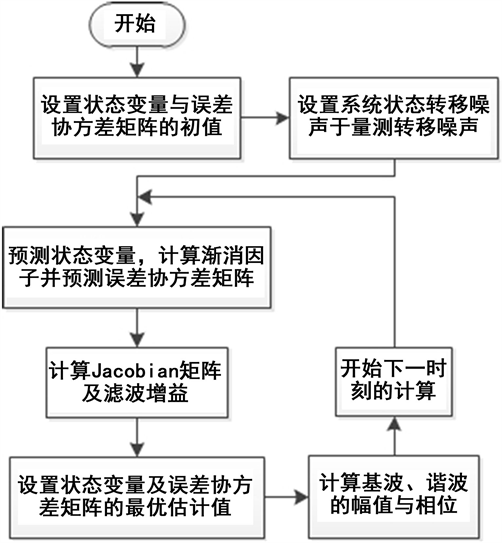Figure 1. Flowchart of transient power quality signal detection based on STEKF

2) 根据(9)式，由状态变量 ${X}_{k}$ 预测 $k+1$ 时刻的状态变量 ${X}_{k+1|k}$ ，并由(16)~(21)式计算渐消因子λk+1，根据(15)式预测 $k+1$ 时刻的误差协方差矩阵。

3) 利用(11)和(12)式分别计算量测转移函数Jacobian矩阵 ${H}_{k}$ 与滤波增益 ${K}_{k+1}$ ，根据(13)和(14)式计算 $k+1$ 时刻状态变量的最优估计值 ${X}_{k+1}$ 以及误差协方差矩阵的最优估计值 ${P}_{k+1}$

4) 由步骤3中得到的状态变量最优估计值 ${X}_{k+1}$ 中的分量，分别计算基波幅值 ${{a}_{1}|}_{k+1}$ 与相位 ${{\phi }_{1}|}_{k+1}$ ，以及各次谐波的幅值 ${{a}_{n}|}_{k+1}$ 与相位 ${{\phi }_{n}|}_{k+1}$

5) 返回本节的步骤2，预测 $k+2$ 时刻的状态变量以及误差协方差矩阵。

5. 仿真分析

${\sigma }_{\text{RMSE}}\left(k\right)=\sqrt{\frac{1}{k}\underset{i=\text{0}}{\overset{k-\text{1}}{\sum }}{\left({c}_{i}-{c}_{{i}_{0}}\right)}^{2}}$ (22)

5.1. 调幅信号的仿真分析

TEKF算法和STEKF算法对调幅信号的仿真情况如图2所示。图2(a)和图2(b)分别为幅值和相位的估计曲线，(c)和(d)分别为幅值和相位的RMSE曲线。由图2可知，STEKF算法仿真得到的幅值和相位曲线与TEKF算法相比更接近于幅值和相位理论值的曲线，从幅值和相位的RMSE曲线可以看出，STEKF的RMSE值明显小于TEKF的RMSE值。表1给出了在t = 0.5 s时两种算法的幅值、相位估计值与误差情况。由表1可知STEKF算法在t = 0.5 s时幅值、相位的绝对误差分别为0.0000 p.u.和0.0002 rad，RMSE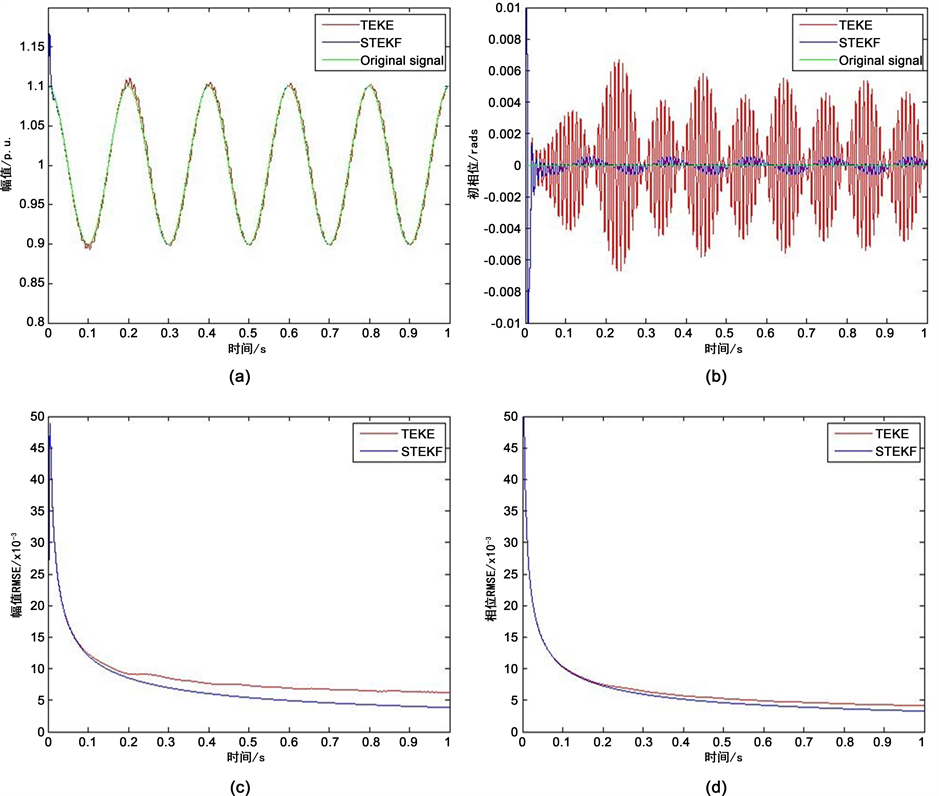Figure 2. Estimation of amplitude & phase of amplitude modulation signalTable 1. Error analysis of amplitude modulation signal (t = 0.5 s)

5.2. 调相信号的仿真分析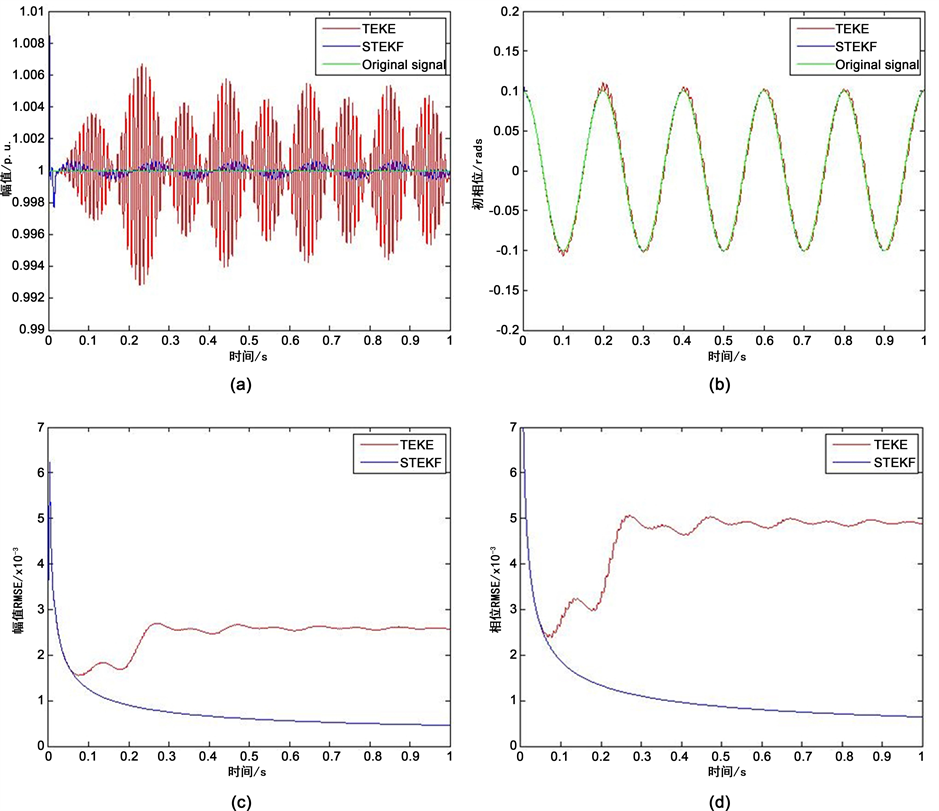Figure 3. Estimation of amplitude & phase of phase modulation signalTable 2. Error analysis of phase modulation signal (t = 0.5 s)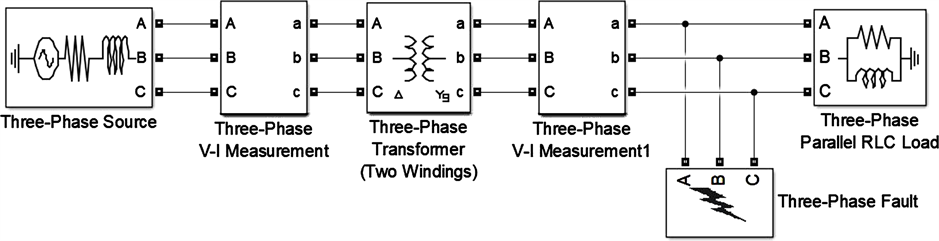Figure 4. Simulation model of fault voltage signal

5.3. 故障电压信号的仿真分析

5.4. 含谐波的电压信号的仿真分析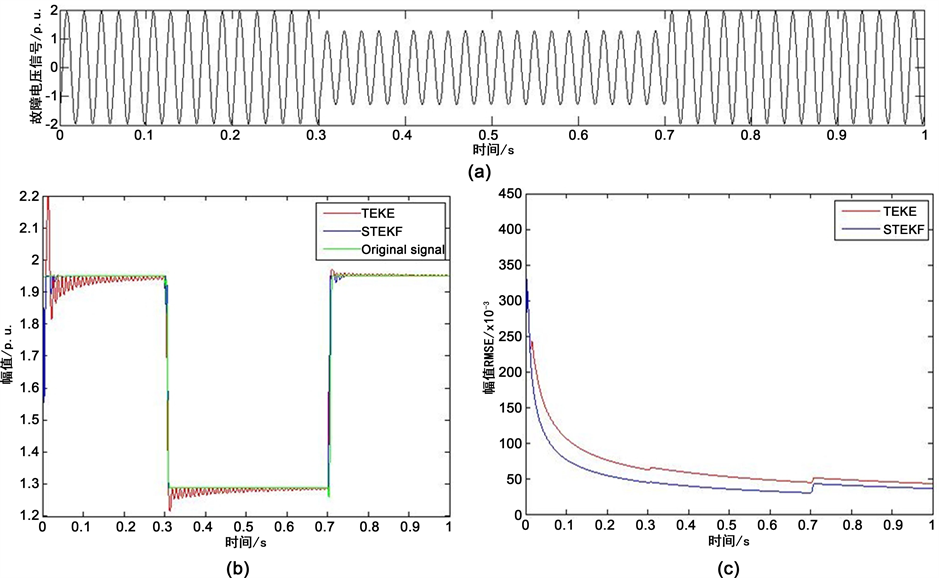Figure 5. Estimation of amplitude of fault voltage signalTable 3. Error analysis of fault voltage signal amplitude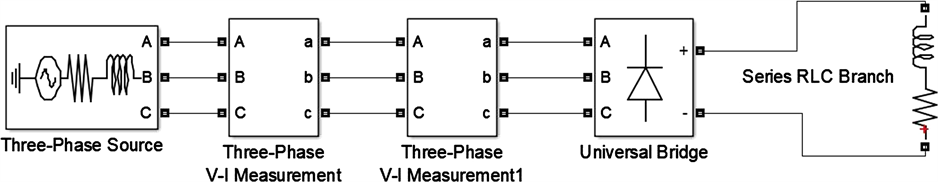Figure 6. Simulation model of voltage signal containing harmonics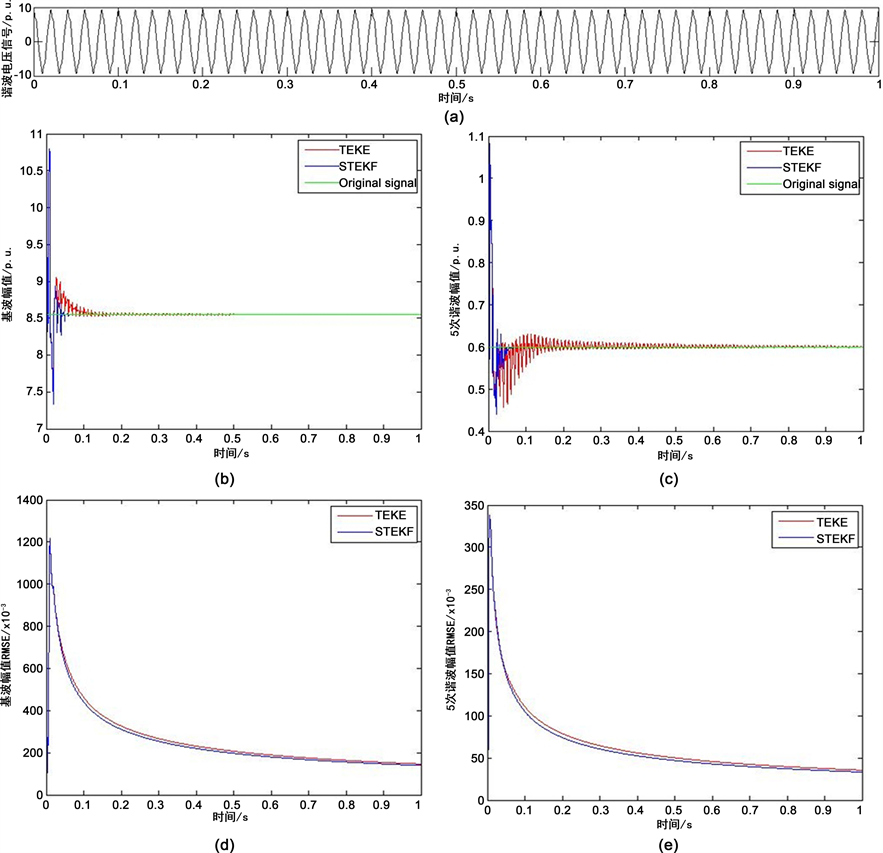Figure 7. Estimation of amplitude of fundamental wave & 5th order harmonicTable 4. Error analysis of voltage signal amplitude containing harmonics

6. 结论

 He, S., Li, K. and Zhang, M. (2014) A New Transient Power Quality Disturbances Detection Using Strong Trace Filter. IEEE Transactions on Instrumentation & Measurement, 63, 2863-2871.
https://doi.org/10.1109/TIM.2014.2326762

 Liu, S.M. and Guo, T. (2016) An Adaptive DFT Algorithm for Measuring Power System Synchrophasors Based on Rectangular Coordinate. Power and Energy Engineering Confer-ence, Brisbane, 15-18 November 2015, 1-5.

 郑恩让, 杨润贤, 高森. 关于电力系统FFT谐波检测存在问题的研究[J]. 电力系统保护与控制, 2006, 34(18): 52-57.

 刘开培, 张俊敏. 基于DFT的瞬时谐波检测方法[J]. 电力自动化设备, 2003, 23(3): 8-10.

 胡海棠, 陆文颖. 谐波检测方法的研究探讨[J]. 电气自动化, 2017, 39(6): 109-111.

 Ning, D., Wei, C., Suo, J., et al. (2009) Voltage Sag Disturbance Detection Based on RMS Voltage Method. Power and Energy Engineering Conference, APPEEC 2009, Asia-Pacific, Wuhan, 27-31 March 2009, 1-4.

 任祖华, 王柏林, 王冰. 基于滑动窗口求取电压均方根值的闪变检测[J]. 电测与仪表, 2013(7): 21-24.

 肖湘宁, 徐永海, 刘昊. 电压凹陷特征量检测算法研究[J]. 电力自动化设备, 2002, 22(1): 19-22.

 Dwivedi, U.D. and Singh, S.N. (2009) Denoising Techniques With Change-Point Approach for Wavelet-Based Power-Quality Moni-toring. IEEE Transactions on Power Delivery, 24, 1719-1727.
https://doi.org/10.1109/TPWRD.2009.2022665

 周龙华, 付青, 余世杰, 等. 基于小波变换的谐波检测技术[J]. 电力系统及其自动化学报, 2010(1): 80-85.

 于静文, 薛蕙, 温渤婴. 基于卡尔曼滤波的电能质量分析方法综述[J]. 电网技术, 2010, 34(2): 97-103.

 李江, 王义伟, 魏超, 张鹏. 卡尔曼滤波理论在电力系统中的应用综述[J]. 电力系统保护与控制, 2014, 42(6): 135-144.

 Ferrero, R., Pegoraro, P.A. and Toscani, S. (2016) Dynamic Fundamental and Harmonic Synchrophasor Estimation by Extended Kalman Filter. IEEE International Work-shop on Applied Measurements for Power Systems, Aachen, 28-30 September 2016, 1-6.

 De la O Serna, J.A. and Rodriguez-Maldonado, J. (2011) Instantaneous Oscillating Phasor Estimates with Taylor Kth Kalman Filters. IEEE Transactions on Power Systems, 26, 2336-2344.
https://doi.org/10.1109/TPWRS.2011.2157539

 周东华, 席裕康. 一种带多重次优渐消因子的扩展卡尔曼滤波器[J]. 自动化学报, 1991, 17(6): 689-695.

 Yin, Z., Li, G., Sun, X., et al. (2016) A Speed Estimation Method for Induction Motors Based on Strong Tracking Extended Kalman Filter. Power Electronics and Motion Control Conference, Hefei, 22-26 May 2016, 798-802.

 Boutayeb, M. and Aubry, D. (1999) A Strong Tracking Extended Kalman Observer for Nonlinear Discrete-Time Systems. IEEE Transac-tions on Automatic Control, 44, 1550-1556.
https://doi.org/10.1109/9.780419

Top